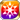# First Winter Holiday

Type
Equipment
Rank
5★
Max level
40
Enhancer cost
2000
Enhancer EXP
20000
Vision Clear Reward
Source
Free (From a Snowman with Love)
Release dates
• JP: 2018-12-13
• GL: 2019-12-26
AlchemistCodeDBFruit cake, stew, a cake decorated with a gothic aesthetic, salad, cheese dogs, onigiri, and best of all...presents! This was the most perfect first Winter Holiday I could have ever had, and it was all thanks to the Phantoms! "What a wonderfully lively event!" I'm so glad that my gift of a handmade hairpiece was well-received, too! All of these festivities can be thoroughly enjoyed, regardless of one's age! (Gratitude of Birgitta)

## Stats

#### Simple stats

TypeLvl 1Lvl 30Lvl 40
HP+20+49+60

LevelHP
1+20
2+21
3+22
4+23
5+24
6+25
7+26
8+27
9+28
10+29
LevelHP
11+30
12+31
13+32
14+33
15+34
16+35
17+36
18+37
19+38
20+39
LevelHP
21+40
22+41
23+42
24+43
25+44
26+45
27+46
28+47
29+48
30+49
LevelHP
31+50
32+51
33+52
34+53
35+54
36+55
37+56
38+57
39+58
40+60

## Group skills

### Ethereal Wall of the Sacred Night

#### Simple stats

TypeLvl 1Lvl 30Lvl 40
All Resist Debuff Res+5+12+15

#### Detailed stats

LevelAll Resist Debuff Res
1+5
2+5
3+5
4+5
5+6
6+6
7+6
8+6
9+7
10+7
LevelAll Resist Debuff Res
11+7
12+7
13+8
14+8
15+8
16+8
17+9
18+9
19+9
20+9
LevelAll Resist Debuff Res
21+10
22+10
23+10
24+10
25+11
26+11
27+11
28+11
29+12
30+12
LevelAll Resist Debuff Res
31+12
32+12
33+13
34+13
35+13
36+13
37+14
38+14
39+14
40+15

#### Limit Break

TypeLB1LB2LB3LB4LB5
All Resist Debuff Res+2+3+5+7+9

### Party Planning!

#### Simple stats

TypeLvl 1Lvl 30Lvl 40
Light Res+2+4+6
AGI+2+4+6

#### Detailed stats

LevelLight ResAGI
1+2+2
2+2+2
3+2+2
4+2+2
5+2+2
6+2+2
7+2+2
8+2+2
9+2+2
10+2+2
LevelLight ResAGI
11+3+3
12+3+3
13+3+3
14+3+3
15+3+3
16+3+3
17+3+3
18+3+3
19+3+3
20+3+3
LevelLight ResAGI
21+4+4
22+4+4
23+4+4
24+4+4
25+4+4
26+4+4
27+4+4
28+4+4
29+4+4
30+4+4
LevelLight ResAGI
31+5+5
32+5+5
33+5+5
34+5+5
35+5+5
36+5+5
37+5+5
38+5+5
39+5+5
40+6+6

#### Limit Break

TypeLB1LB2LB3LB4LB5
Light Res+1+1+2+3+4
AGI+1+1+2+3+4

### Silent Night, Holy Night

#### Simple stats

TypeLvl 1Lvl 30Lvl 40
HP+60+164+200
MATK+17+41+50
Strong vs Snow+7+20+25

#### Detailed stats

LevelHPMATKStrong vs Snow
1+60+17+7
2+63+17+7
3+67+18+7
4+70+19+8
5+74+20+8
6+77+21+9
7+81+22+9
8+85+22+10
9+88+23+10
10+92+24+11
LevelHPMATKStrong vs Snow
11+95+25+11
12+99+26+12
13+103+27+12
14+106+28+13
15+110+28+13
16+113+29+13
17+117+30+14
18+121+31+14
19+124+32+15
20+128+33+15
LevelHPMATKStrong vs Snow
21+131+33+16
22+135+34+16
23+138+35+17
24+142+36+17
25+146+37+18
26+149+38+18
27+153+39+19
28+156+39+19
29+160+40+19
30+164+41+20
LevelHPMATKStrong vs Snow
31+167+42+20
32+171+43+21
33+174+44+21
34+178+44+22
35+182+45+22
36+185+46+23
37+189+47+23
38+192+48+24
39+196+49+24
40+200+50+25

#### Limit Break

TypeLB1LB2LB3LB4LB5
HP+24+48+72+96+120
MATK+6+12+18+24+30
Strong vs Snow+3+6+9+12+15

#### Max Limit Break

TypeLB5
Dark+8
Strong vs Snow+10

## Vision abilities

### Limit Reached Bonus

#### Ability base

• Slot:Support

Revealed after Limit Reached. Raises AGI after appearing on map.

#### Ability max

• Slot:Action

Raises AGI after appearing on map.